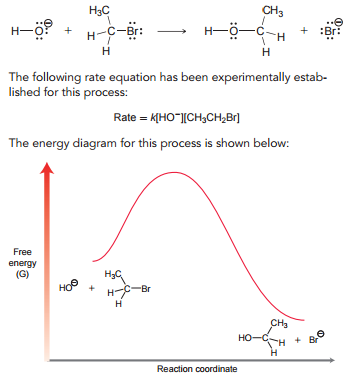Problem: Consider the following reaction:(c) Would you expect this process to be exothermic or endothermic? Explain.

FREE Expert Solution
90% (279 ratings)
Problem Details

Consider the following reaction:

(c) Would you expect this process to be exothermic or endothermic? Explain.What scientific concept do you need to know in order to solve this problem?

Our tutors have indicated that to solve this problem you will need to apply the Enthalpy concept. You can view video lessons to learn Enthalpy Or if you need more Enthalpy practice, you can also practice Enthalpy practice problems .

What is the difficulty of this problem?

Our tutors rated the difficulty of Consider the following reaction:(c) Would you expect this pr... as medium difficulty.

What professor is this problem relevant for?

Based on our data, we think this problem is relevant for Professor Dubey's class at UCF.

What textbook is this problem found in?

Our data indicates that this problem or a close variation was asked in . You can also practice practice problems .